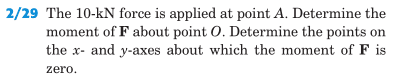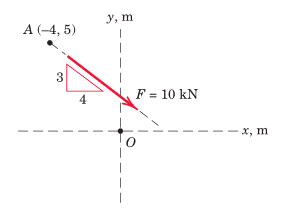# Moments question

## Homework Statement## Homework Equations

tau = r x F

varignons theorem

## The Attempt at a Solution

I drew the diagram and the moment arm method and the component of the force perpendicular to vector OA seemed hard to find, so I used varignons theorem.

tau = -abs(5000*5)+abs(6000*-4) = -16000 (which seems to be correct)

I don't understand the second part of the question however.
Is it asking me to find new points for the axis of rotation (one on the y axis and one on the x axis) such that the moment about each point is zero? The answers suggest that I'm just finding the x and y intercepts.

e.g. if I find some point on the y axis, won't the x component of the force always cause a clockwise rotation, and the y component won't cause any rotation because it is parallel; so how can the moment be zero?